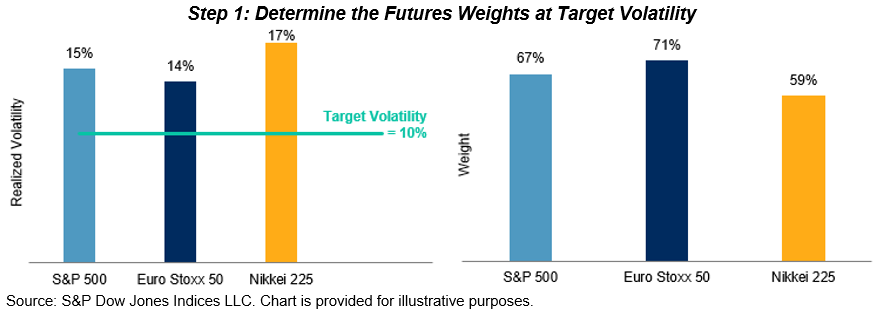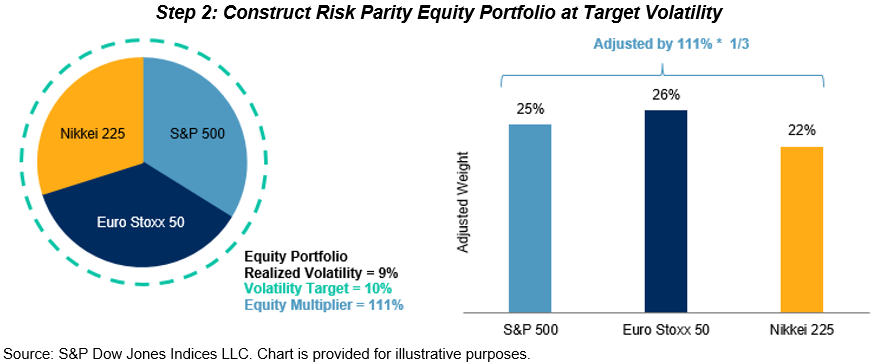# The S&P Risk Parity Indices: Methodology

In earlier posts, we analyzed the historical performance, risk contribution versus capital allocation, and return attribution and leverage of the S&P Risk Parity Indices. The results demonstrate that this indices in this series could potentially serve as benchmarks to measure the performance of active risk parity strategies. In this post, we will dig deeper into the methodology and walk through our rationale behind the index rules.

Differences in risk parity strategies arise from the asset classes (and instruments) chosen in the strategy, the risk measurement used, and the handling of the assets’ risk contribution to the portfolio. To build a transparent risk parity benchmark, we use a bottom-up approach that employs long-term realized volatility to allocate across asset classes.

• Underlying Asset Classes: The underlying asset classes are equity, fixed income, and commodities, as tracked by futures contracts.
• Liquidity: For all futures contracts used, each has a minimum annual total dollar value traded of USD 5 billion to ensure replicability and tradability.
• Risk Measurement: We use long-term realized volatility to measure risk. The look-back window has a minimum of a five-year history at the beginning of our back-tested period and is capped at 15 years as we accumulate more data. We use realized volatility rather than forecast volatility to avoid dependency on volatility forecasting models.
• Weighting Mechanism: We target an equal amount of risk contribution from each asset class to the overall portfolio volatility. In order to do this, we calculate the position weight simply as the pre-defined target volatility divided by the long-term realized volatility for each asset class. Due to correlation among asset classes, the realized volatility of the risk parity portfolio would usually be lower than the target volatility. We then apply a leverage factor to achieve the target volatility. Within each asset class, futures are combined using the same approach to ensure equal risk contribution from futures to the asset class they belong to.
• Rebalancing Frequency: The indices calculate target weights at month-end and apply them on the second trading day of the next month.

Here is a hypothetical example that illustrates the index construction process of the S&P Risk Parity Index – 10% Target Volatility (TV). In particular, we show how the weight of the S&P 500® futures is determined.

Hypothetical Weighting of the S&P Risk Parity – 10% TV

Suppose the long-term realized volatility of the S&P 500 futures contract is 15%. To reach the target volatility of 10%, we need to allocate 10%/15% = 67% to it and the rest to cash.Next, we calculate the long-term realized volatility of the equity asset class using weights calculated in step 1. Since there are three futures in the equity asset class, we need to divide their weights by three to construct the equity portfolio.

Suppose the realized volatility of equities is 9%. To reach the target volatility of 10%, we need to apply a multiplier of 10%/9% = 111% to the three constituent futures contract within the asset class. As a result, the weight of the S&P 500 futures in the equity asset class is set to 67% * 1/3 * 111% = 25%.Finally, we calculate long-term realized volatility of the portfolio using weights calculated in step 2. Since there are three asset classes in the equity asset class, we need to divide their weights by three to construct the multi-asset portfolio.

Let’s say the portfolio’s realized volatility turns out to be 8%. To reach the target volatility of 10%, we need to apply a multiplier of 10% / 8% = 125% to the 26 constituent futures contract. As a result, the weight of the S&P 500 futures is finalized as 25% * 1/3 * 125% = 10%.The S&P Risk Parity Indices are constructed in a rules-based, transparent manner using tradable, liquid instruments to facilitate implementation. As we have seen in other parts of the blog series, the indices track the composite performance of active risk parity funds much closer than a traditional 60/40 equity/bond portfolio.